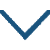秋夜将晓出篱门迎凉有感

• qiū
• jiāng
• xiǎo
• chū
• mén
• yíng
• liáng
• yǒu
• gǎn
•
• sòng
•
• yóu
• sān
• wàn
• dōng
• hǎi
•
• qiān
• rèn
• yuè
• shàng
• tiān
•
• mín
• lèi
• jìn
• chén
•
• nán
• wàng
• wáng
• shī
• yòu
• nián
•

译文

三万里长的黄河奔腾向东流入大海，
五千仞高的华山耸入云霄上摩青天。
中原人民在胡人压迫下眼泪已流尽，
他们盼望官军收失地盼了一年又一年。

注释

三万里：长度，形容它的长，是虚指。河：指黄河。
五千仞（rèn）：形容它的高。仞，古代计算长度的一种单位，周尺八尺或七尺，周尺一尺约合二十三厘米。岳：指五岳之一西岳华山。黄河和华山都在金人占领区内。一说指北方泰、恒、嵩、华诸山。摩天：迫近高天，形容极高。摩，摩擦、接触或触摸。
遗民：指在金占领区生活的汉族人民，却认同南宋王朝统治的人民。泪尽：眼泪流干了，形容十分悲惨、痛苦。胡尘：指金人入侵中原，也指胡人骑兵的铁蹄践踏扬起的尘土和金朝的暴政。胡，中国古代对北方和西方少数民族的泛称。
南望：远眺南方。王师：指宋朝的军队。

赏析

“河”，指黄河，哺育中华民族的母亲；岳，指东岳泰山、中岳嵩山、西岳华山等立地擎天的峰柱。巍巍高山，上接青冥；滔滔大河，奔流入海。两句一横一纵，北方中原半个中国的形胜，鲜明突兀、苍莽无垠地展现在我们眼前。奇伟壮丽的山河，标志着祖国的可爱，象征着民众的坚强不屈，已给读者以丰富的联想。然而，如此的山河，如此的人民，却长期以来沦陷在金朝贵族铁蹄蹂躏之下，下两句笔锋一转，顿觉风云突起，诗境向更深远的方向开拓。
“泪尽”一词，千回万转，中原广大人民受到压迫的沉重，经受折磨历程的长久，企望恢复信念的坚定不移与迫切，都充分表达出来了。他们年年岁岁盼望着南宋能够出师北伐，可是岁岁年年此愿落空。当然，他们还是不断地盼望下去。人民的爱国热忱真如压在地下的跳荡火苗，历久愈炽；而南宋统治集团则正醉生梦死于西子湖畔，把大好河山、国恨家仇丢在脑后，可谓心死久矣，又是多么可叹！后一层意思，在诗中虽未明言点破，强烈的批判精神则跃然可见。• chuán
• guā
• zhōu
•
• sòng
•
• wáng
• ān
• shí
• jīng
• kǒu
• guā
• zhōu
• shuǐ
• jiān
•
• zhōng
• shān
• zhǐ
• shù
• chóng
• shān
•
• chūn
• fēng
• yòu
• 绿
• jiāng
• nán
• àn
•
• míng
• yuè
• shí
• zhào
• huán
•• chū
• sài
•
• táng
•
• wáng
• chāng
• líng
• qín
• shí
• míng
• yuè
• hàn
• shí
• guān
•
• wàn
• cháng
• zhēng
• rén
• wèi
• huán
•
• dàn
• shǐ使
• lóng
• chéng
• fēi
• jiàng
• zài
•
• jiào
• yīn
• shān
•• xiàng
•
• táng
•
• liú
• zhū
• què
• qiáo
• biān
• cǎo
• huā
•
• xiàng
• kǒu
• yáng
• xiá
•
• jiù
• shí
• wáng
• xiè
• táng
• qián
• yàn
•
• fēi
• xún
• cháng
• bǎi
• xìng
• jiā
•• mán
•
• shū
• jiāng
• 西
• zào
• kǒu
•
• sòng
•
• xīn
• tái
• xià
• qīng
• jiāng
• shuǐ
•
• zhōng
• jiān
• duō
• shǎo
• xíng
• rén
• lèi
•
• 西
• běi
• wàng
• cháng
• ān
•
• lián
• shù
• shān
•
• qīng
• shān
• zhē
• zhù
•
• jìng
• dōng
• liú
•
• jiāng
• wǎn
• zhèng
• chóu
•
• shān
• shēn
• wén
• zhè
•• zèng
• huā
• qīng
•
• táng
•
• jǐn
• chéng
• guǎn
• fēn
• fēn
•
• bàn
• jiāng
• fēng
• bàn
• yún
•
• zhǐ
• yīng
• tiān
• shàng
• yǒu
•
• rén
• jiān
• néng
• huí
• wén
•微信公众号